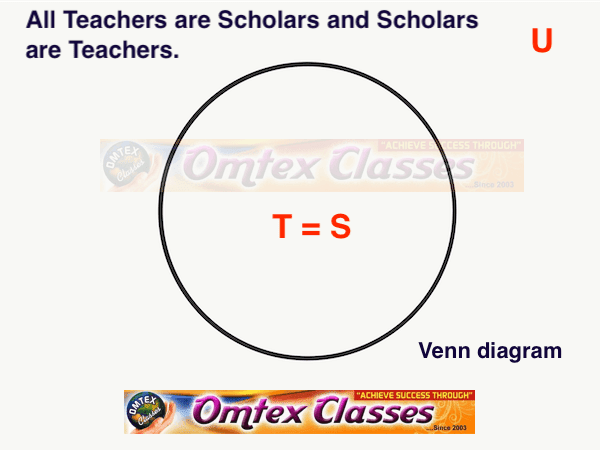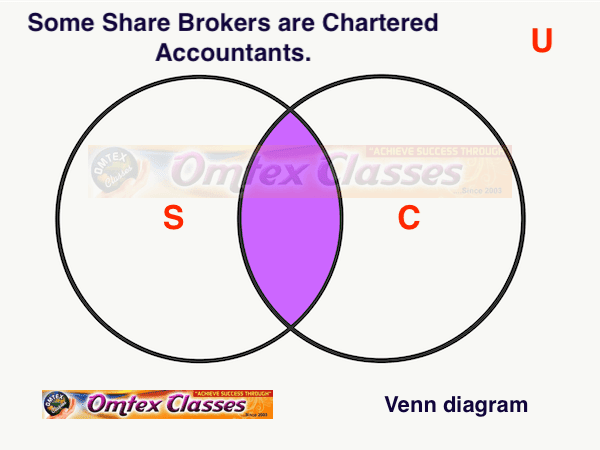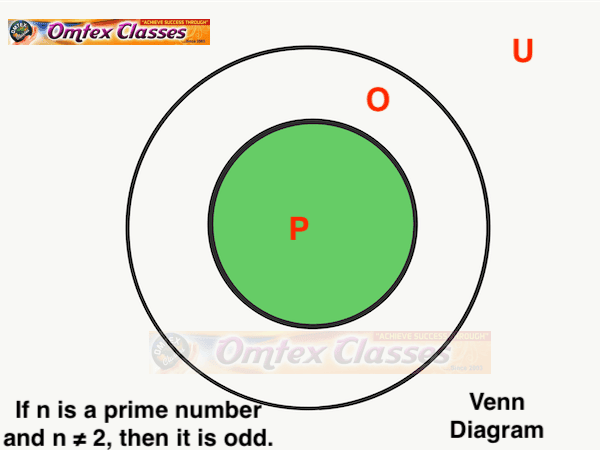SSC BOARD PAPERS IMPORTANT TOPICS COVERED FOR BOARD EXAM 2024

### Mathematical Logic Exercise 1.10 [Pages 22 - 27] Balbharati solutions for Mathematics and Statistics 1 (Commerce) 12th Standard HSC Maharashtra State Board Chapter 1

EXERCISE 1.10 [PAGES 22 - 27]

### Balbharati solutions for Mathematics and Statistics 1 (Commerce) 12th Standard HSC Maharashtra State Board Chapter 1 Mathematical Logic Exercise 1.10 [Pages 22 - 27]

EXERCISE 1.10Q 1.1  PAGE 27
Exercise 1.10 | Q 1.1 | Page 27

Represent the truth of the following statement by the Venn diagram.

Some hardworking students are obedient.

#### SOLUTION

Let U : The set of all students.
H : The set of all hardworking students.
O : The set of all obedient students.

The above Venn diagram represents truth of the given statement, H ∩ O ≠ φ
EXERCISE 1.10Q 1.2  PAGE 27
Exercise 1.10 | Q 1.2 | Page 27

Represent the truth of the following statement by the Venn diagram.

No circles are polygons.

#### SOLUTION

Let U : The set of all closed geometrical figures in plane.
P : The set of all polygons
C : The set of all circles.The above Venn diagram represents truth of the given statement, P ∩ C ≠ φ

EXERCISE 1.10Q 1.3  PAGE 27
Exercise 1.10 | Q 1.3 | Page 27

Represent the truth of the following statement by the Venn diagram.

All teachers are scholars and scholars are teachers.

#### SOLUTION

Let U : The set of all human beings.
T : The set of all teachers.
S : The set of all scholarsThe above Venn diagram represents truth of the given statement, T = S

EXERCISE 1.10Q 1.4  PAGE 27
Exercise 1.10 | Q 1.4 | Page 22

Represent the truth of the following statement by the Venn diagram.

If a quadrilateral is a rhombus, then it is a parallelogram.

#### SOLUTION

Let U : The set of all quadrilaterals.
P : The set of all parallelograms.
R : The set of all rhombuses.The above Venn diagram represents truth of the given statement, R ⊂ P.

EXERCISE 1.10Q 2.1  PAGE 27
Exercise 1.10 | Q 2.1 | Page 27

Draw a Venn diagram for the truth of the following statement.

Some share brokers are chartered accountants.

#### SOLUTION

Let U : The set of all human beings.
S : The set of all share brokers.
C : The set of all chartered accountants.The above Venn diagram represents the truth of the given statement i.e., S ∩ C ≠ φ.

EXERCISE 1.10Q 2.2  PAGE 27
Exercise 1.10 | Q 2.2 | Page 27

Draw a Venn diagram for the truth of the following statement.

No wicket keeper is bowler, in a cricket team.

#### SOLUTION

Let U : The set of all human beings.
W : The set of all wicket keepers.
B : The set of all bowlers.The above Venn diagram represents the truth of the given statement i.e., W ∩ B = φ.

EXERCISE 1.10Q 3.1  PAGE 27
Exercise 1.10 | Q 3.1 | Page 27

Represent the following statement by the Venn diagram.

Some non-resident Indians are not rich.

#### SOLUTION

Let, U : The set of all human beings.
N : The set of all non-resident Indians.
R : The set of all rich people.The above Venn diagram represents the truth of the given statement i.e., N - R ≠ φ

EXERCISE 1.10Q 3.2  PAGE 27
Exercise 1.10 | Q 3.2 | Page 27

Represent the following statement by the Venn diagram.

No circle is rectangle.

#### SOLUTION

Let, U : The set of all geometrical figures.
C : The set of all circles.
R : The set of all rectangles.The above Venn diagram represents the truth of the given statement i.e., C ∩ R = φ.

EXERCISE 1.10Q 3.3  PAGE 27
Exercise 1.10 | Q 3.3 | Page 27

Represent the following statement by the Venn diagram.

If n is a prime number and n ≠ 2, then it is odd.

#### SOLUTION

Let, U : The set of all real numbers.
P : The set of all prime numbers n and n ≠ 2.
O : The set of all odd numbers.The above Venn diagram represents the truth of the given statement i.e., P ⊂ O.